﻿ Correlation between Engineering Stress-Strain and True Stress-Strain Curve

### Correlation between Engineering Stress-Strain and True Stress-Strain CurveOPEN ACCESSPEER-REVIEWED

## Correlation between Engineering Stress-Strain and True Stress-Strain Curve

Iman Faridmehr1, Mohd Hanim Osman1,, Azlan Bin Adnan2, Ali Farokhi Nejad2, Reza Hodjati1, Mohammadamin Azimi1

1Faculty of Civil Engineering, Universiti Teknologi, Malaysia, Skudai, Johor. Malaysia

2Faculty of Mechanical Engineering, Universiti Teknologi, Malaysia, Skudai, Johor. Malaysia

### Abstract

The most commonly accepted method in evaluation of the mechanical properties of metals would be the tension test. Its main objective would be to determine the properties relevant to the elastic design of machines and structures. Investigation of the engineering and true Stress-strain relationships of three specimens in conformance with ASTM E 8 – 04 is the aim of this paper. For the purpose of achieving this aim, evaluation of values such as ultimate tensile strength, yield strength, percentage of elongation and area reduction, fracture strain and Young's Modulus was done once the specimens were subjected to uniaxial tensile loading. The results indicate that the properties of steel materials are independent from their thickness and they generally yield and fail at the same stress and strain values. Also, it is concluded that the maximum true stress values are almost 15% higher than that of the maximum engineering stress values while the maximum true strain failure values are 1.5% smaller than the maximum engineering strain failure values.

### At a glance: Figures

1234
Prev Next

• Faridmehr, Iman, et al. "Correlation between Engineering Stress-Strain and True Stress-Strain Curve." American Journal of Civil Engineering and Architecture 2.1 (2014): 53-59.
• Faridmehr, I. , Osman, M. H. , Adnan, A. B. , Nejad, A. F. , Hodjati, R. , & Azimi, M. (2014). Correlation between Engineering Stress-Strain and True Stress-Strain Curve. American Journal of Civil Engineering and Architecture, 2(1), 53-59.
• Faridmehr, Iman, Mohd Hanim Osman, Azlan Bin Adnan, Ali Farokhi Nejad, Reza Hodjati, and Mohammadamin Azimi. "Correlation between Engineering Stress-Strain and True Stress-Strain Curve." American Journal of Civil Engineering and Architecture 2, no. 1 (2014): 53-59.

 Import into BibTeX Import into EndNote Import into RefMan Import into RefWorks

### 1. Introduction

The significant role of the mechanical properties in evaluation of the fundamental properties of engineering materials along with development of new materials and also quality control of materials for application in design and construction cannot be neglected. Once a material is meant to be applied within an engineering structure that will be subject to loading, it must be guaranteed that the material possesses enough strength and rigidity to withstand the loads it will experience in its service life. Consequently, a number of experimental techniques have been developed by engineers for the purpose of mechanical testing of engineering materials subjected to tension, compression, bending or torsion loading. The tension test would be the most common type of test incorporated in measurement of the mechanical properties of materials. Provision of basic design information on materials strength is the purpose of conduction of tension test. Also, tension test is considered as an acceptance test for the specification of materials. Major parameters such as the tensile strength (UTS), yield strength or yield point (σy), elastic modulus (E), percent elongation (ΔL%) and the reduction in area (RA%) are obtained during the tension test in order to describe the stress-strain curve. Other parameters including toughness, resilience and Poisson’s ratio (ʋ) can also be calculated through the use of this testing technique. Conduction of the tensile test is done through application of longitudinal or axial load at a specific extension rate to a standard tensile specimen with known dimensions, including the gauge length and cross sectional area perpendicular to the load direction, until failure. In order to calculate the stress and strain, the applied tensile load and extension are recorded during the test.

A range of universal standards provided by professional societies such as American Society of Testing and Materials ASTM E8 , British standard , JIS standard  and DIN standard  provide tests based on preferential purposes. Each standard may contain a variety of test standards suitable for different materials, dimensions and fabrication histories. For instance, ASTM E8  is a standard test method for tension testing of metallic materials and ASTM B557  is a standard test method of tension testing and also for casting aluminum and magnesium alloy products.

### 2. Research Methodology

2.1. Test Specimens

Figure 1 illustrates the preparation of a standard specimen in a round or a square section along the gauge length in conformance with ASTM E8 . For the specimens being firmly gripped during testing, sufficient length and a surface condition must be provided for both ends of the specimens. Table 1 demonstrates the standardized initial length Lo which varies with the diameter (Do) or the cross-sectional area (Ao) of the specimen. This is due to the fact that the % elongation might be underestimated if the gauge length is too long in this case . For the purpose of producing the final specimen to be tested, any kind of heat treatments should be applied on the test specimen prior to machining. This was done to avoid surface oxide scales that could behave as stress concentration which could eventually affect the final tensile properties as a result of premature failure. Some exceptions including the surface hardening or surface coating on the materials might exist. Application of these processes must be done after specimen machining to gain the tensile properties results that entail the actual specimen surface conditions.

#### Table 1. Dimensional Relationships of Tensile Specimens Used in Different Countries

The specimens selected for this study were machined in conformance with ASTM (?) with a thickness of 6mm, 8mm and 10mm respectively as shown in Figure 2.

2.2. Test Equipment

The equipment applied for the conduction of tensile testing includes both simple devices and complicated controlled systems. The most commonly used equipment for this test would be the so-called universal testing machines driven by mechanical screw or hydraulic systems. A relatively simple screw-driven machine incorporating two screws for the purpose of applying the load along with a hydraulic testing machine incorporating the pressure of oil in a piston to supply the load are illustrated in Figure 3. Application of these types of machines is not only for tension, but also for compression, bending and torsion tests. A variety of loads, strain, or testing machine motions (stroke) are provided by a more modernized closed-loop servo-hydraulic machine through the use of a combination of actuator rod and piston. The majority of the machines used nowadays in tests are connected to a computer-controlled system that could graphically display the load and extension data along with the stress and strain calculations. Extensometer is another equipment incorporated in this test. An extensometer is defined as a device applied to measure changes in the length of an object used in tensile tests and stress-strain measurements. Finally, the device used to measure the distance between two opposite sides of an object is called a caliper.

The universal testing machine incorporated in this test belongs to to DARTEC Company with capacity 4000 KN in compression and 5000 KN in tension. The standard ASTM E4-1998 was used to calibrate the machine with the ambient temperature is 26.0°C ± 2°C. Figure 4 illustrates the equipment used in this test including the testing machine, extensometer and caliper respectively from left to right.

2.3. Test Procedure

The specimens used in this test are made of low carbon steel with a thickness of 6mm, 8mm and 10mm respectively. First, the dimensions of the specimens (gauge length, thickness and width) are tabulated in Table 2 in order to determine the engineering stress and engineering strain values. Then, the location of the gauge length was marked along the parallel length of each specimen for subsequent necking observations and strain measurements. Next, the specimen was fit on to the Universal Testing Machine (UTM) and then, the extensometer was installed on the specimen. After that the testing began and load and extension values were recorded. Finally, the following calculations were done to determine the material characteristics.

2.3.1. Stress and Strain Relationship

Once an external tensile loading is applied on a specimen, elastic and plastic deformations will be anticipated. Initially, an elastic deformation will occur to the metal resulting in a linear relationship of load and extension. Calculation of the engineering stress and engineering strain will be done using these two parameters for the purpose of yielding a relationship as shown in Figure 5 incorporating equations 1 and 2 as follows: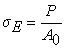(1)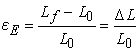(2)

Where

σE is the engineering stress.

εE is the engineering strain.

P is the external axial tensile load.

Ao is the original cross-sectional area of the specimen.

Lo is the original length of the specimen.

Lf is the final length of the specimen.

The dimension of the engineering stress is Pascal (Pa) or N/m2 based on the SI Metric Unit while the "psi" (pound per square inch) dimension could also be incorporated as well. Once the elastic deformation is in progress, the engineering stress-strain relationship follows the Hook's Law and slope of the curve demonstrates the Young's modulus (E).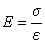(3)

The significance of the Young's modulus calculation would be in determination of the materials deflection in engineering applications. For instance, deflection of the structural beams is known to be a critical factor in designing the engineering components or structures including buildings, bridges, ships and etc. Also, spring constants or Young's modulus values are required for tennis racket and golf club applications.

2.3.2. Yield Strength and Ultimate Tensile Strength

Through consideration of the stress-strain curve beyond the elastic limit, yielding will occur at the beginning of plastic deformation if the tensile loading continues. Calculation of the yield stress, σy, is done through dividing the load at yielding (Py) by the original cross-sectional area of the specimen (Ao) as demonstrated in equation 4.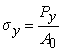(4)

The yield point could be located directly from the load-extension curve of the metals like steel, particularly low carbon ones, or iron or in molybdenum and polycrystalline titanium (Figure 6). The yield point elongation phenomenon indicates the upper yield point followed by a sudden stress or load reduction until the lower yield point is reached. Extending of the specimen is continued at the yield point elongation without a substantial change in the stress level. Figure 3 indicates the yield strength determination at 0.2% offset or 0.2% strain which is carried out through drawing a straight line parallel to the slope of the stress-strain curve in the linear section, reaching the intersection on the x-axis at a strain equal to 0.002. An interception among the 0.2% offset line and the stress strain curve indicates the yield strength at 0.2% offset or 0.2% strain. The yield strength which is an indication of the plastic deformation onset is known to be a significant factor in engineering structural or component designs where application of safety factors are common (equation 5). Several considerations are taken into account for safety factors including the deterioration estimation, structural components and the consequences of failed structures such as loss of life, economical losses and etc. Generally, a safety factor of 2 is required for buildings, which is quite low since the calculation of loads has been well understood. A safety factor of 2 for automobiles along with safety factors of 3 - 4 for pressure vessels are two examples for this issue.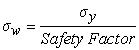(5)

Beyond yielding, an increase in the stress for permanent deformation of the specimen occurs through continuous loading. At this time, the specimen would be strain hardened or work hardened. Through application of continuous loading, the maximum point will be reached for the stress-strain curve, which is the ultimate tensile strength (σTS) (equation 6). At this stage, the highest stress before necking could be beard by the specimen. This is observed through a local reduction in the cross sectional area of the specimen commonly seen in the center of the gauge length.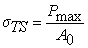(6)

2.3.3. True Stress and True strain

Stress has units of a force measure divided by the square of a length measure, and the average stress on a cross-section in the tensile test is clearly the applied force divided by the cross-sectional area. In a similar manner, an approximation is made on the strain component along the long axis of the specimen as the change in length divided by the original, reference length. This might seem simple at first glance; however, some choices are still left to be made. For instance, which are must be chosen for the cross-sectional area? Which are should be used, the original one or the current one? Also, should length changes be compared against the original length of the specimen? The answer lies in the fact that several types of stress and stress measurements are done according to our methodology. Fixed reference quantities are used to distinguish the engineering stress and strain, mainly the original cross-sectional area or original length. Such definitions are accurate in most of the engineering applications due to fixed values of the cross-sectional area and length of the specimen while the loads are applied. In other circumstances such as the tensile test, a substantial change in the cross-sectional area and the length of the specimen is expected. In such occasions, the engineering stress determined using the above definition (as the ratio of the applied load to the undeformed cross-sectional area) seems to be an inaccurate measure. Hence, alternative stress and strain measurement methods are available to overcome this issue. The following lines are dedicated to the true stress and true strain discussion.

The ratio of the applied load to the instantaneous cross-sectional area is the definition of the true stress. There could exist a relationship between the true stress and engineering stress once no volume change is assumed in the specimen. Under this assumption;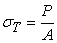(7)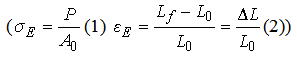Where,

A is the reduced cross-sectional area of the specimen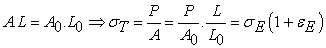(8)

True strain is defined as the instantaneous increase rate in the instantaneous gauge length defined as true strain.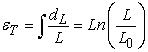(9)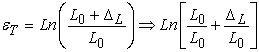(10)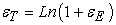(11)

In practice, it is noteworthy to mention that the true stress and strain are basically indistinguishable from the engineering stress and strain at small deformations (Figure 8). Yet, it should be noted that the true stress could be much larger than the engineering stress once the strain increases and the consequently, the cross sectional of the specimen decreases.

2.3.4. Fracture Strength and Strain

Plastic deformation is not uniform after necking and hence, a decrease in the stress occurs accordingly until fracture. Calculation of the fracture strength (σ fracture) could be done from the load at fracture divided by the original cross-sectional area, Ao, as shown in equation 11. The fracture strain, εf, is defined as the equivalent strain at fracture strength. Drawing a straight line starting at the fracture point of the stress-strain curve parallel to the slope in the linear relation would give the fracture strain of the specimen. The fracture strain is indicated through the interception of the parallel line at the x axis.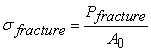(12)

2.3.5. Tensile Ductility

The percentage of elongation or percentage reduction in area represents the tensile ductility of the specimen as shown in the following equations: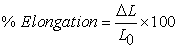(13)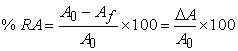(14)

Where,

Af is the cross-sectional area of specimen at fracture.

### 3. Results and Discussion

The material characteristics for all the three specimens are demonstrated in Table 3. Also, a pictorial view of the failed specimens is demonstrated in Figure 9 and finally, the engineering and true stress-strain curve are drawn for the three specimens used in the tests in Figure 10. According to the stress-strain curves used in this study, the specimen behaves having a definite spring constant according to the so-called Hooke’s Law (Eq.3). The stress-strain curve is linear in this “elastic” region. The yield point is defined as the point where the linearity ends. In the stress-strain curve, “E” acts as the slope of the loading line in the elastic region. As long as loading of the metal is done within the elastic region, the strains are totally recoverable and the specimen will return to its original dimensions as the load is relaxed to zero. Once the load value exceeds the corresponding yield point, gross plastic deformation, which is permanent, will occur to the specimen even the load is returned to zero afterwards. According to the stress-strain curves obtained from the tests in this study, it is evident that the low-carbon steel specimens have a definite yield point (Figure 10). Yet, determination of the offset yield strength was also done through drawing a parallel line to the elastic portion of the curve, starting from the 0.2% strain level. The comparison between the two yield points from direct observation and the yield offset method revealed similar results. Furthermore, the ultimate tensile strength values of the three tested specimens in Table 3 show a 3% difference among these values while the elongation and fracture strain values are almost equal.

### 4. Conclusions

The Universal Test was conducted on the three test specimens made of low carbon steel with a thickness of 6mm, 8mm and 10mm respectively and a loading rate of 5 mm/min at the Laboratory of Structures and Materials, Universiti Teknologi Malaysia (UTM). Based on the results, it was concluded that the thickness of the specimens had no effect on the properties of steel materials mainly yield strength and Young module and yielding and failure of such specimens occurs at the same stress and strain values. Also, the maximum engineering stress values appeared to be 15% lower than that of the maximum true stress values while the maximum engineering strain failure values are 1.5% higher than the maximum true strain failure values.

### Acknowledgements

The material required for the specimen fabrication and financial assistance was provided by Universiti Teknologi Malaysia (UTM).

### References

  Standard Test Methods for Tension Testing of Metallic Materials, D.o. Defense., Editor August 2013, American Society of Testing and Materials (ASTM)In article  Metallic materials - Tensile testing, July 2001, BRITISH STANDARD.In article  Japanese Industrial Standards (JIS), J.S.A. (JSA), Editor 2005.In article  Standard Test Methods for Mechanical Testing of Steel Products-Metric ASTM A 1058b, 2012, DIN Deutsches Institut für Normung e. V.In article  Standard Test Methods for Tension Testing Wrought and Cast Aluminum- and Magnesium-Alloy Products, A.S.f.T.a. Materials, Editor 2010, DIN Deutsches Institut für Normung e. V.In article  Roylance, D., Stress-strain curves. Massachusetts Institute of Technology study, Cambridge, 2001.In article